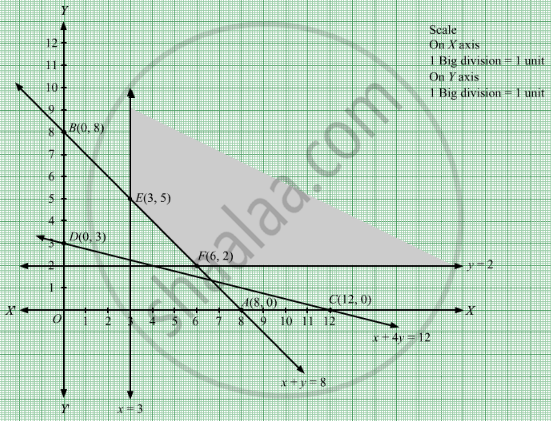# Minimize Z = 2x + 4y Subject to X + Y ≥ 8 X + 4 Y ≥ 12 X ≥ 3 , Y ≥ 2 - Mathematics

Sum

Minimize Z = 2x + 4y
Subject to

$x + y \geq 8$
$x + 4y \geq 12$
$x \geq 3, y \geq 2$

#### Solution

First, we will convert the given inequations into equations, we obtain the following equations:
x + y = 8, x + 4y = 12, x = 3, y = 2

Region represented by x + y ≥ 8:
The line x + y = 8 meets the coordinate axes at A(8, 0) and B(0, 8) respectively. By joining these points we obtain the line x + y = 8.
Clearly (0,0) does not satisfies the inequation x + y ≥ 8. So,the region in xy plane which does not contain the origin represents the solution set of the inequation x + y ≥ 8.

Region represented by x + 4y ≥ 12:
The line x + 4y = 12 meets the coordinate axes at C(12, 0) and D(0, 3) respectively. By joining these points we obtain the line x + 4y = 12.
Clearly (0,0) satisfies the inequation x + 4y ≥ 12. So,the region in xy plane which contain the origin represents the solution set of the inequation x + 4y ≥ 12.

The line x = 3 is the line that passes through the point (3, 0) and is parallel to Y axis.≥ 3 is the region to the right of the line x = 3.

The line y = 2 is the line that passes through the point (0, 12) and is parallel to X axis.≥ 2 is the region above the line y = 2.The corner points of the feasible region are E(3, 5) and F(6, 2).

The values of Z at these corner points are as follows.

 Corner point Z = 2x + 4y E(3, 5) 2 × 3 + 4 × 5 = 26 F(6, 2) 2 × 6 + 4 × 2 = 20

Therefore, the minimum value of Z is 20 at the point F(6, 2). Hence, x = 6 and y =2 is the optimal solution of the given LPP.
Thus, the optimal value of Z is 20.

Concept: Graphical Method of Solving Linear Programming Problems
Is there an error in this question or solution?

#### APPEARS IN

RD Sharma Class 12 Maths
Chapter 30 Linear programming
Exercise 30.2 | Q 10 | Page 32